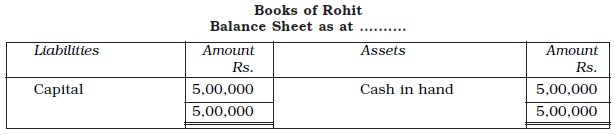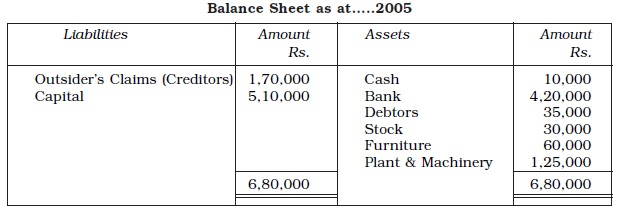Study Materials: CBSE Notes

Our CBSE Notes for Notes 3. Recording of transaction-I - Accounting Equation | Class 11 Accounts - Toppers Study is the best material for English Medium students cbse board and other state boards students.

Notes ⇒ Class 11th ⇒ Accounts ⇒ 3. Recording of transaction-I

# Notes 3. Recording of transaction-I - Accounting Equation | Class 11 Accounts - Toppers Study

Topper Study classes prepares CBSE Notes on practical base problems and comes out with the best result that helps the students and teachers as well as tutors and so many ecademic coaching classes that they need in practical life. Our CBSE Notes for Notes 3. Recording of transaction-I - Accounting Equation | Class 11 Accounts - Toppers Study is the best material for English Medium students cbse board and other state boards students.

## Notes 3. Recording of transaction-I - Accounting Equation | Class 11 Accounts - Toppers Study

CBSE board students who preparing for class 11 ncert solutions maths and Accounts solved exercise chapter 3. Recording of transaction-I available and this helps in upcoming exams 2023-2024.

### You can Find Accounts solution Class 11 Chapter 3. Recording of transaction-I

• All Chapter review quick revision notes for chapter 3. Recording of transaction-I Class 11
• NCERT Solutions And Textual questions Answers Class 11 Accounts
• Extra NCERT Book questions Answers Class 11 Accounts
• Importatnt key points with additional Assignment and questions bank solved.

Chapter 3 Accounts class 11

### Accounting Equation class 11 Accounts Chapter 3. Recording of transaction-I

• Notes 3. Recording Of Transaction-I - Accounting Equation | Class 11 Accounts - Toppers Study
• Class 11 Ncert Solutions
• Solution Chapter 3. Recording Of Transaction-I Class 11
• Solutions Class 11
• Chapter 3. Recording Of Transaction-I Accounting Equation Class 11

## Notes 3. Recording of transaction-I - Accounting Equation | Class 11 Accounts - Toppers Study

Accounting Equation :

Total Assets = Total Liabilities

Or

Total Assets = Internal Liabilities + External Liabilities

Or

Total Assets = Capital + Liabilities

Accounting equation signifies that the assets of a business are always equal to the total of its liabilities and capital (owner’s equity).

A = L + C
Where,
A = Assets
L = Liabilities
C = Capital

Now,

(i) A – L = C
(ii) A – C = L

The accounting equation depicts the fundamental relationship among
the components of the balance sheet, it is also called the Balance Sheet Equation.

⇒ The balance sheet is a statement of assets, liabilities and capital.

⇒ The claim of the proprietors is called capital and that of the outsides is known as liabilities.

⇒ Each element of the equation is the part of balance sheet, which states the financial position of the business on a particular date.

⇒ When we analyse the transactions, we actually try to know that how balance sheet of a business entity gets affected.

⇒ Asset side of the balance sheet is the list of assets, which the business entity owns.

⇒ The liabilities side of the balance sheet is the list of owner’s claims and outsider’s claims, i.e., what the business entity owes.

⇒ The equality of the assets side and the liabilities side of the balance sheet is an undeniable fact and this justifies the name of accounting equation as balance sheet equation also.

#### Classification of Transactions:

Following are the nine basic transactions:

(1) Increase in assets with corresponding increase in capital.

(2) Increase in assets with corresponding increase in liabilities.

(3) Decrease in assets with corresponding decrease in capital.

(4) Decrease in assets with corresponding decrease in liabilities.

(5) Increase and decrease in assets.

(6) Increase and decrease in liabilities

(7) Increase and decrease in capital

(8) Increase in liabilities and decrease in capital

(9) Increase in capital and decrease in liabilities.

Example: Rohit started business with a capital of Rs. 5,00,000.

Solution:From the accounting point of view we see:

(i) The resources of this business entity is in the form of cash, i.e., Rs. 5,00,000.

(ii) Sources of this business entity is the contribution by Rohit (Proprietor) Rs. 5,00,000 as Capital .

"In the above balance sheet, the total assets are equal to the liabilities of the business."

Example 1.
1. Opened a bank account in State Bank of India with an amount of
Rs. 4,80,000.

Analysis of transaction: This transaction increases the cash in hand
(assets) and decreases cash (asset) by Rs. 4,80,000.
2. Bought furniture for Rs. 60,000 and cheque was issued on the same day.
Analysis of transaction: This transaction increases furniture (assets) and
decreases bank (assets) by Rs. 60,000.
3. Bought plant and machinery for the business for Rs. 1,25,000 and an advance of Rs. 10,000 in cash is paid to M/s Ramjee Lal.
Analysis of transaction: This transaction increases plant and machinery
(assets) by Rs. 1,25,000, decreases cash by Rs. 10,000 and increases
liabilities (M/s Ramjee lal as creditor)by Rs. 1,15,000.

4. Goods purchased from M/s Sumit Traders for Rs. 55,000.
Analysis of transaction: This transaction increases goods (assets) and
increases liabilities (M/s Sumit Traders as creditors) by Rs. 55,000.
5. Goods costing Rs. 25,000 sold to Rajani Enterprises for Rs. 35,000.
Analysis of transaction: This transaction decreases stock of goods (assets) by Rs. 25,000 and increases assets (Rajani Enterprises as debtors Rs. 35,000) and capital (with the profit of Rs. 10,000)

Solution:In terms of accounting equation
A = L + C
Rs. 6,80,000 = Rs. 1,70,000 + Rs. 5,10,000

Important Study materials for classes 06, 07, 08,09,10, 11 and 12. Like CBSE Notes, Notes for Science, Notes for maths, Notes for Social Science, Notes for Accountancy, Notes for Economics, Notes for political Science, Noes for History, Notes For Bussiness Study, Physical Educations, Sample Papers, Test Papers, Mock Test Papers, Support Materials and Books.Mathematics Class - 11th

NCERT Maths book for CBSE Students.

books

## Study Materials List:

##### Notes ⇒ Class 11th ⇒ Accounts
1. Introduction to Accounting
2. Theory Base of Accounting
3. Recording of transaction-I
4. Recording of transaction-II
5. Bank Reconciliation Statement
6. Trial Balance and Rectification of Errors
7. Depreciation, Provisions and Reserves

New Books